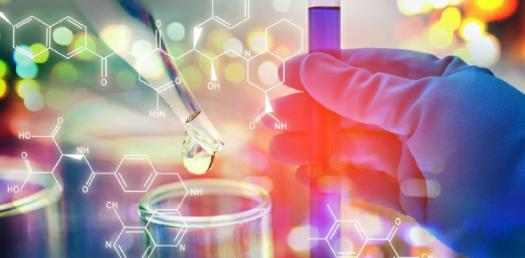# The Ultimate Quiz On Chemistry For 10th Grade

10 Questions | Total Attempts: 125SettingsThe word atom comes from the ancient Greek word atomos meaning unsplittableor uncuttable. A philospher called Democrites carried out a thought experiment. He imagined taking a piece of rock and hitting it with a hammer. That's enough history, let's do Chemistry!

• 1.
The numbers in the options below represent the masses of the three sub-atomic particles. Pick the correct combination.
• A.

Proton 1 Neutron 0 Electron 1

• B.

Proton 0 Neutron 1 Electron 1

• C.

Proton 1 Neutron 1 Electron 0

• D.

Proton 1 Neutron 1 Electron 1

• 2.
The numbers in the options below represent the masses of the three sub-atomic particles. Pick the correct combination.
• A.

Proton 1 Neutron 0 Electron 1

• B.

Proton 0 Neutron 1 Electron 1

• C.

Proton 1 Neutron 1 Electron 0

• D.

Proton 1 Neutron 1 Electron 1

• 3.
The electrons are found...
• A.

In the nucleus of the atom

• B.

In orbit around the outside of the nucleus

• C.

Dotted throughout the atom

• D.

In lines radiating out from the nucleus

• 4.
The neutrons of an atom are found...
• A.

In the nucleus of the atom

• B.

In orbit around the outside of the nucleus

• C.

Dotted throughout the atom

• D.

In lines radiating out from the nucleus

• 5.
Carbon has two isotopes. These atoms have...
• A.

The same number of protons, but different numbers of electrons

• B.

The same number of electrons, but different numbers of protons

• C.

The same number of protons, but different numbers of neutrons

• D.

The same number of neutrons, but different numbers of electrons

• 6.
If an element has 56 protons, 56 electrons and 81 neutrons, what is its mass number?
• A.

56

• B.

81

• C.

137

• D.

112

• 7.
The protons of an atom are found...
• A.

In the nucleus of the atom

• B.

In orbit around the outside of the nucleus

• C.

Dotted throughout the atom

• D.

In lines radiating out from the nucleus

• 8.
Pick the correct combination for the charges of sub-atomic particles.
• A.

Proton +ve Neutron 0 Electron -ve

• B.

Proton 0 Neutron +ve Electron -ve

• C.

Proton +ve Neutron -ve Electron 0

• D.

Proton 0 Neutron 0 Electron -ve

• 9.
The atomic number of an element is 11 and its mass number is 23. Pick the correct combination of protons, neutrons and electrons.
• A.

P=12, n=12, e=11

• B.

P=11, n=11, e=12

• C.

P=12, n=11, e=11

• D.

P=11, n=12, e=11

• 10.
The mass number of an element tells us...
• A.

The number of neutrons in the nucleus

• B.

The number of electrons orbiting the nucleus

• C.

The number of protons orbiting the nucleus

• D.

The number of electrons in the nucleus

Related TopicsBack to top